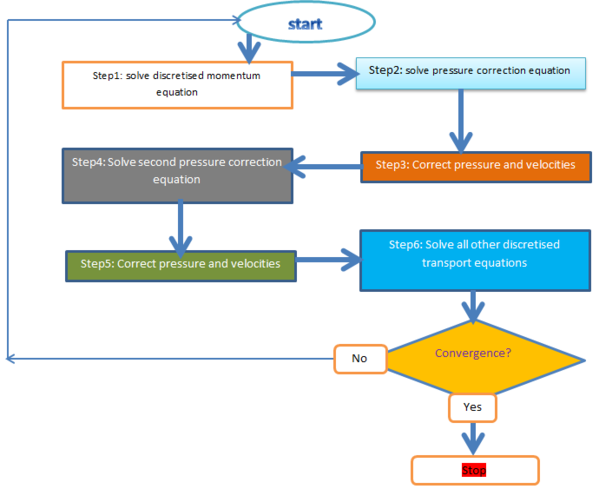# 3. Solver¶

The solver is devided into two parts, the first part of the script includes the Files called outside of the main loop. Then there is the second part of the solver which is the loop where the equations are solved with the PIMPLE method.

## 3.1. The files called outside the main¶

• fvCFD.H : collection of fundamental tools for performing finite volume calculation

• singlePhaseTransportModel.H : a simple single-phase transport model based on the viscosity model

• turbulentTransportModel.H : type definition of the turbulence model for incompressible flows, there are three models available:

• LAMINAR -> direct simulation, Navier-Stockes equations are solved on the all calculation domain (no turbulence model)
• RAS -> uses Reynolds Averadged simulation modelling
• LES -> uses large eddy simulation modelling
• fvOptions.H : provides a base set of controls (source type, start time, duration,…). Mainly used to add source terms to equations of the mathematical model (for instance the coriolis force).

• pimpleControl.H : supplies convergence information/checks for the PIMPLE loop.

## 3.2. The files called inside the main¶

• postProcess.H : executes application FUNCTIONOBJECT to post-process.
• createMesh.H
• createControl.H : Control of the PISO algorithm
• createFields.H
• createFvOptions.H
• createTimeControls.H : Read the control parameters used by setDeltaT
• CourantNo.H : calculate mean and maximu Courant number.
• setInitialDeltaT.H : sets initial time step.
• initContinuityErrs.H : Declares and initiates the cumulative continuity error.

All these files are not specific to the coriolis case, they are found in almost every OpenFOAM solver.

Most of the constants and parameters declared in these files are not to be changed within these files. You should change them in the system folder of the tutorials (Dict files) which will then change the .H files.

## 3.3. The loop and the files called in the loop¶

### 3.3.1. The files called in the loops¶

UEqn.H : Contains the momentum equation which is the equation solved to obtain the velocity field.

rhokEqn.H : Contains the equation solved for the density which is the internal energy equation.

pEqn.H : Contains the equation solved for the pressure. It is simply the divergence of the momentum equation.

### 3.3.2. The solver loop¶

Coriofoam code uses the PISO method combined to the SIMPLE method (PIMPLE). Basically it is a SIMPLE loop nested in a PISO loop. This is an explanatory scheme of the PISO method :source image

The coriofoam solver uses 3 time-loops. In the second one the momentum equation (file UEqn.H called) and the density equation (file rhokEqn.H called) are solved. Whereas, the pressure equation is only solved in the third time loop (correction).

## 3.4. Momentum equation with Boussinesq approximation¶

The version of the momentum equation solved is the version with the boussinesq approximation.

Boussinesq approximation :

• incompressible flow
• density and temperature do not vary much overall around their mean values

Rotating terms have to appear in our Navier-Stockes equation : The coriolis force and the driving force.

velocity Equations (UEqn) $$D_{t}\vec{u}=-\vec{\bigtriangledown}\dfrac{p}{\rho_0}+\nu\Delta\vec{u}+\dfrac{\rho ' \vec{g}}{\rho_0}-\Omega²\vec{r}-2\vec{\Omega}\wedge\vec{u}$$

In this equation, we have the classic Navier-stockes equation, but also the coriolis force $$Fc=-2\Omega\vec{u}$$ the driving force $$Fe=-\Omega²\vec{r}$$ And the flotability term $$\dfrac{\rho ' \vec{g}}{\rho_0}=-b \vec{e_z}$$

The pressure equation is found by taking the divergence of the momentum equation :

$$\Delta p=-\rho (\bigtriangledown (u \cdot \bigtriangledown\ u )-\Omega²\bigtriangledown r-2\bigtriangledown(\vec{\Omega}\wedge\vec{u}))$$ is the equation that we have after simplifications

The pressure and the velocity equations are coupled, that’s why we use the PISO method.

Then we have the rhô equation, taking into account the flotability term :

$$D_{t} (b)= K\Delta b$$

The incompressible property give us the following equation :

$$\dfrac{\partial \rho}{\partial t}+\vec{\bigtriangledown} \left(\rho \vec{u}\right)= 0$$

All these equations are solved or used in the PISO algorithm, defined in the file Coriofoam.C

## 3.5. Important parameters of the loop¶

The important control parameters of the loop are defined and can be changed in the system folder. Especially in the Dict files located in 0_init, constant and system. These folders can be found in the tutorials part.